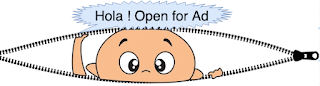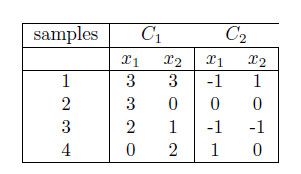## Latest Posts

Sample Data:-  Use following data set 1 and data set-2 for classifier using least square approachData Set -1Data Set -2

Problem statement
:- Sample program to find the linear classifier using least square approach.

```import sys
import matplotlib.pyplot as plt
import pylab as pl
import numpy as np

def getMultiplePoints(x,y,weight,boundX1,boundX2):
x1 =[x,0]
x2 =[0,y]
pointsX = []
pointsY = []
pointsX.insert(1,y)
pointsX.insert(2,0)
pointsY.insert(1,0)
pointsY.insert(2,x)
#for boundX1
pointsX.insert(0,boundX1)
temp = -(weight*boundX1 + weight)/weight
pointsY.insert(0,temp)
#for boundX2
pointsX.insert(3,boundX2)
temp = -((weight*boundX2) + weight)/weight
pointsY.insert(3,temp)
return (pointsX,pointsY)

#plot points
def getCoordinatesList(dataset,weightPlot):
XList1 =[]
YList1 =[]
XList2 =[]
YList2 =[]
count = 0
boundX = -4
boundY =  4
#compute classifier co-ordinates
x1 = - (weightPlot/weightPlot)
y1 = 0
x2 = 0
y2 = - (weightPlot/weightPlot)
itr = len(dataset)/2
# compute some random point with slope as W and bias b
plotTup = getMultiplePoints(x1,y2,weightPlot,boundX,boundY)
for row in dataset:
if(count< itr):
XList1.append(row)
YList1.append(row)
else:
XList2.append(row)
YList2.append(row)
count = count+1
return (XList1, YList1,XList2, YList2,plotTup)

def plotDataPointsAndClassifier(plotData,weightPlotLS):
boundX = -4
boundY =  4
colorLS = 'black'
(XList1, YList1,XList2, YList2,plotTupLS) =
getCoordinatesList(plotData,weightPlotLS)
#Draw points with red and Blue color
plt.plot(XList1, YList1, 'ro',XList2, YList2, 'bo')
plt.axis([boundX, boundY, boundX, boundY])
plt.plot(plotTupLS,plotTupLS,color = colorLS,label='Least Square')
plt.legend(loc='best')
plt.show()

def compute(row, weights):
bias = weights
output = bias
for i in range(len(row)-1):
output += weights[i] * row[i]
if row == 1 and output > 0:
return True
elif row == -1 and output <= 0:
return True
else:
return False

#compute b to such data data point are segrated
def getB(dataset,weights):
flag = True
epoch = 1
while(True):
flag = False ; epoch = epoch + 1
for row in dataset:
prediction = compute(row, weights)
if not prediction:
weights = row -(weights*row+weights*row)
flag = True
if epoch == 10 or flag == False :
break
return weights

# To find classifier Minimum Squared Error Procedures - using Pseudoinverse
def LeastSquareClassifier(inputData):
#Compute b based on input size. B is 1x<size> matrix with 1
size = len(inputData)
b = [1 for x in range(size)]
#find b's transpose - > 8x1 matrix
bt = np.matrix(b).getT()
#Prepare input matrix from dataset
m = np.matrix(inputData)
#find tranpose of input matrix
t = m.getT()
#Multiply transpose of input matrix and matrix -  (Y^tY)
mul = t*m
#find inverse of outcome of above operation - (Y^tY)^-1
inv = mul.getI()
# Find pseudo inverse- Multiply inversed matrix with
#transpose of input matrix - (Y^tY)^-1Y^t
secondMul = inv * t
#find solution matrix - Multiply pseudo matrix with b
f = secondMul * bt
#compute weight for ploting classifier
weightPlot = []
weightPlot.insert(0,f.item(1))
weightPlot.insert(1,f.item(2))
weightPlot.insert(2,f.item(0))
return weightPlot

def ClassifierOnTable1():
#Find least square classifier weight
inputData = [[1,3,3], [1,3,0],[1,2,1] ,[1,0,2] ,
[-1,1 ,-1],[-1,0, 0],[-1,1,1],[-1,-1 ,0]]
plotData =  [[3,3,1], [3,0,1],[2,1,1] ,[0,2,1] ,[-1 ,1,-1],
[0, 0,-1],[-1,-1,-1],[1,0,-1]]
# find classifier for given dataset and Plot it.
weightPlotLS = LeastSquareClassifier(inputData)
#plot data points and classifier
plotDataPointsAndClassifier(plotData,weightPlotLS)

def ClassifierOnTable2():
inputData = [[1,3,3], [1,3,0],[1,2,1] ,[1,0,1.5] ,
[-1,1 ,-1],[-1,0, 0],[-1,1,1],[-1,-1 ,0]]
plotData  = [[3,3,1], [3,0,1],[2,1,1] ,[0,1.5,1] ,[-1 ,1,-1],
[0, 0,-1],[-1,-1,-1],[1,0,-1]]
# find classifier for given dataset and Plot it.
weightPlotLS = LeastSquareClassifier(inputData)
#plot data points and classifier
plotDataPointsAndClassifier(plotData,weightPlotLS)

# map the inputs to the function blocks
options = {
1 : ClassifierOnTable1,
2 : ClassifierOnTable2,
}
#start
if __name__ == '__main__':
Dataset1C1 = [ [3,3], [3,0],[2,1] ,[0,1.5]]
Dataset1C2 = [[-1 ,1],[0, 0],[-1,-1],[1 ,0]]
print "1. LeastSquareClassifier on Data points in Table 1 \n \
2. LeastSquareClassifier) on Data points in Table 2 \n"
num = int(raw_input())
options[num]()
```

Sample Output
:-
[zytham@s158519-vm perceptron]\$ python LSP.py
1. LeastSquareClassifier on Data points in Table 1
2. LeastSquareClassifier) on Data points in Table 2

1.